#### 期刊菜单

Airborne PD Radar’s Sea Clutter Emulation Based on K Distribution
DOI: 10.12677/JISP.2018.74028, PDF, HTML, XML, 下载: 809  浏览: 2,038

Abstract: When airborne radar radiates to the sea, targets’ echoes follow with sea clutter of much more power, and the work of sea clutter emulation does much help to the suppress of sea clutter. Sea clutter’s intensity is stochastically changing in time and space with some relativity. In order to simulate sea clutter’s characteristic simulation, K distribution is selected to achieve the simulation of sea clutter’s intensity’s fluctuant characteristic, while cubic power spectrum is selected to carry out relativity characteristic simulation with Doppler frequency effect introduced by wave and platform moving. Emulation result shows that the built model could reflect fluctuant characteristic and relativity characteristic of sea clutter perfectly.

1. 引言

2. 海杂波K分布起伏模型

$K\left(x;a;v\right)=\frac{2}{a\Gamma \left(v+1\right)}{\left(\frac{x}{2a}\right)}^{v+1}{K}_{v}\left(\frac{x}{a}\right),\text{\hspace{0.17em}}x>0,v>-1$ (1)

3. 机载脉冲多普勒雷达功率谱模型

3.1. 高斯功率谱模型

$S\left(f\right)={S}_{0}\mathrm{exp}\left(-\frac{{f}^{2}}{2{\sigma }_{f}^{2}}\right)$ (2)

$S\left(f\right)=S{}_{0}\mathrm{e}xp\left[-\frac{{\left(f-\stackrel{¯}{{f}_{d}}\right)}^{2}}{2{\sigma }_{f}^{2}}\right]$ (3)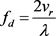(4)

$S\left(f\right)={S}_{0}\mathrm{exp}\left[-\frac{{\left(f-\stackrel{¯}{{f}_{d}}-{f}_{d}\right)}^{2}}{2{\sigma }_{f}^{2}}\right]$ (5)

3.2. 全极点功率谱模型

$S\left(f\right)={S}_{0}\frac{1}{1+{\left(f/{f}_{c}\right)}^{n}}$ (6)

$S\left(f\right)={S}_{0}\frac{1}{1+{\left[\left(f-\stackrel{¯}{{f}_{d}}-{f}_{d}\right)/{f}_{c}\right]}^{n}}$ (7)

4. 相关K分布海杂波模型模拟仿真

4.1. 相关K分布海杂波模型建立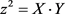(8)

1) 根据仿真需求，选择合适杂波功率谱函数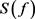，本文采用的杂波功率谱为立方谱函数，即式(7)中n取3后的结果。对 $S\left(f\right)$ 进行N次采样得到功率谱序列 $\left\{S\left(k\right)\right\}$$k=1,2,\cdots ,N$

2) 对得到的功率谱序列 $\left\{S\left(k\right)\right\}$ 进行逆傅里叶变换，得到所求杂波的自相关系数序列 $\left\{s\left(j\right)\right\}$$j=1,2,\cdots ,N$

3) 根据杂波相关系数 $\left\{s\left(j\right)\right\}$ 利用最小二乘法计算得出图1中相关高斯随机序列 $\left\{{w}_{n,i}\right\}$ ( $n=1,2,\cdots ,\theta$$i=1,2,\cdots ,N$ )的相关系数 ${r}_{j}$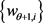$\left({w}_{\theta +2,i}\right)$ ( $i=1,2,\cdots ,N$ 的相关系数 ${q}_{j}$$j=1,2,\cdots ,N$ )。

4) 根据得到的相关系数 ${r}_{j}$${q}_{j}$ ，利用AR模型设计图1中滤波器 ${H}_{1}\left(\omega \right)$${H}_{2}\left(\omega \right)$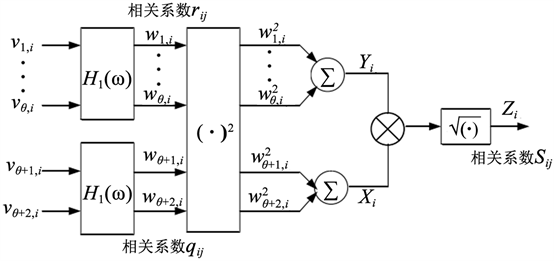Figure 1. Block diagram of correlated K distribution clutter

5) 产生独立的高斯分布序列 $\left\{{v}_{j,i}\right\}$ (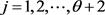)，并经过线性滤波器 ${H}_{1}\left(\omega \right)$${H}_{2}\left(\omega \right)$ 调制产生出满足相应谱特性的相关高斯随机序列 $\left\{{w}_{j,i}\right\}$ (  )。

6) 根据图(1)所示步骤，得到所需要的相关K分布随机序列 $\left\{{z}_{i}=\sqrt{{x}_{i}{y}_{i}}\right\}$ (  )，其中：

${x}_{i}={w}_{\theta +1,i}^{2}+{w}_{\theta +2,i}^{2}$ (9)

${y}_{i}={w}_{1}^{2}+{w}_{2}^{2}+\cdots +{w}_{\theta ,i}^{2}$ (10)

4.2. 相关K分布海杂波模型仿真分析

1) 海杂波功率谱模型选择立方谱模型，3 dB截止频率 ${f}_{c}=90\text{\hspace{0.17em}}\text{Hz}$ ，海杂波平均多普勒频率用 $\stackrel{¯}{{f}_{d}}=30\text{\hspace{0.17em}}\text{Hz}$

2) 雷达飞机平台相对海面的径向速度 ${v}_{r}=100\text{\hspace{0.17em}}\text{m}/\text{s}$ ，雷达工作波长 $\lambda =0.1\text{\hspace{0.17em}}\text{m}$ ，平台运动引起的多普勒频移为 ${f}_{d}=\frac{2{v}_{r}}{\lambda }=2000\text{\hspace{0.17em}}\text{Hz}$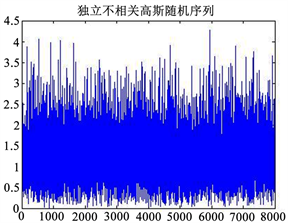Figure 2. Simulation of independent irrelevant random sequences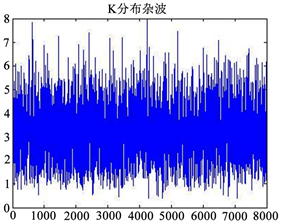Figure 3. K distribution sea clutter generated by simulation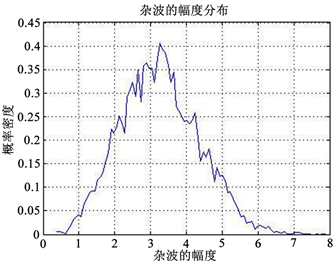Figure 4. Statistical results of sea clutter amplitude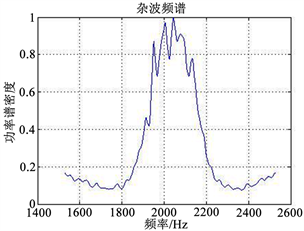Figure 5. Calculation results of sea clutter power spectrum

5. 结束语

  黄斌. 机载海杂波建模与仿真[D]: [硕士学位论文]. 西安: 西安电子科技大学. 2009.  何友, 关键, 彭应宁. 雷达自动检测与恒虚警处理[M]. 北京: 清华大学出版社. 2003.  Conte, E., De Maio, A. and Galdi, C. (2004) Statistical Analysis of Real Clutter at Different Range Resolutions. IEEE Transactions on Aerospace and Electronic Systems, 40, 903-918. https://doi.org/10.1109/TAES.2004.1337463  曹艳霞, 李国君. 基于K分布舰载雷达海杂波仿真方法研究[J]. 雷达科学与技术, 2016, 14(1): 86-90.  丁昊, 李建忠, 安昕, 等. 实测海杂波数据的多普勒谱特性[J]. 雷达科学与技术, 2012, 10(4): 400-408.  常磊. 雷达杂波模拟与应用研究[D]: [硕士学位论文]. 西安: 西安电子科技大学, 2010.  吕世芳. 地、海雷达杂波的模拟技术研究[D]: [硕士学位论文]. 无锡: 江南大学, 2012.